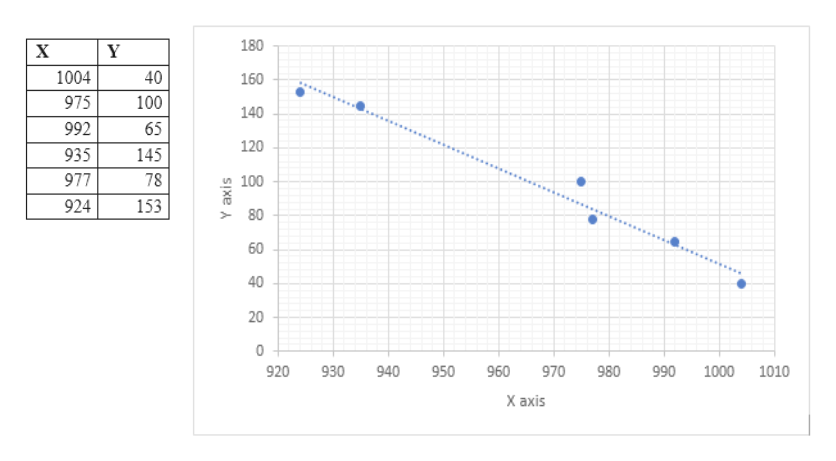Can a low barometer reading be used to predict maximum wind speed of an approaching tropical cyclone? For a random sample of tropical cyclones, let x be the lowest pressure (in millibars) as a cyclone approaches, and let y be the maximum wind speed (in miles per hour) of the cyclone.x1004975992935977924y401006514578153(a) Make a scatter diagram of the data and visualize the line you think best fits the data.  Flash Player version 10 or higher is required for this question. You can get Flash Player free from Adobe's website. (b) Would you say the correlation is low, moderate, or strong? lowmoderate   strongWould you say the correlation is positive or negative? positivenegative    (c) Use a calculator to verify that     x = 5807,     x2 = 5,625,235,     y = 581,     y2 = 66,343 and     xy = 555,293. Compute r. (Round your answer to three decimal places.)  As x increases, does the value of r imply that y should tend to increase or decrease? Explain your answer. Given our value of r, y should tend to increase as x increases.Given our value of r, y should tend to remain constant as x increases.    Given our value of r, y should tend to decrease as x increases.Given our value of r, we can not draw any conclusions for the behavior of y as x increases.

Question

Can a low barometer reading be used to predict maximum wind speed of an approaching tropical cyclone? For a random sample of tropical cyclones, let x be the lowest pressure (in millibars) as a cyclone approaches, and let y be the maximum wind speed (in miles per hour) of the cyclone.

 x 1004 975 992 935 977 924 y 40 100 65 145 78 153
(a) Make a scatter diagram of the data and visualize the line you think best fits the data.

Flash Player version 10 or higher is required for this question.
You can get Flash Player free from Adobe's website.

(b) Would you say the correlation is low, moderate, or strong?
low
moderate
strong

Would you say the correlation is positive or negative?
positive
negative

(c) Use a calculator to verify that
x = 5807,  x2 = 5,625,235,  y = 581,  y2 = 66,343
and
xy = 555,293.

Compute r. (Round your answer to three decimal places.)

As x increases, does the value of r imply that y should tend to increase or decrease? Explain your answer.
Given our value of ry should tend to increase as x increases.
Given our value of ry should tend to remain constant as x increases.
Given our value of ry should tend to decrease as x increases.
Given our value of r, we can not draw any conclusions for the behavior of y as x increases.
Step 1

In this question we have the data, using which we have to plot the scatter plot and best line of fit, also we have to explain the type of correlation exists between the variables. Then some measures have been given in the question using which we have to find the correlation by putting the values in the formula.

Step 2

Part A)

The scatter plot and best fit of line.help_outlineImage Transcriptionclose180 X Y 40 1004 160 975 100 140 992 65 120 935 145 977 78 100 924 153 80 40 20 0 920 930 940 950 960 970 980 990 1000 1010 X axis 60 Y axis fullscreen
Step 3

Part B)

The correlation between the variable X and   Y seems to be strongly negative, this is simply because as we are increasing the values of X the value of y d...

Want to see the full answer?

See Solution

Want to see this answer and more?

Our solutions are written by experts, many with advanced degrees, and available 24/7

See Solution
Tagged in

Other